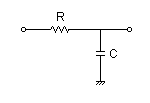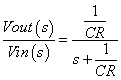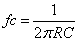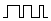# RC Low-pass Filter Design Tool

This page is a web application that design a RC low-pass filter. Use this utility to calculate the Transfer Function for filters at a given frequency or values of R and C. The response of the filter is displayed on graphs, showing Bode diagram, Nyquist diagram, Impulse response and Step response.

## Calculate the transfer function for low-pass filter with R and C values

 Vin(s)→→Vout(s)R=Ω C=F
p:pico, n:nano, u:micro, k:kilo, M:mega

### Frequency analysis

Bode diagram
Phase  Group delay
Nyquist diagram
Pole, zero
Phase margin
Oscillation analysis

Upper and lower frequency limits:
f1= - f2=[Hz] (frequency limits are optional)

### Transient analysis

Step response
Impulse response
Overshoot
Final value of the step response

Simulation time:
0 - [sec] (optional)

## Calculate the R and C values for the filter at a given frequency

 Vin(s)→→Vout(s)fc=Hz C=F (Omitted C=10, 1, 0.1, 0.01…) p:pico, n:nano, u:micro, k:kilo, M:mega Select Resistor Sequence: E24 E12 E6 E96

### Frequency analysis

Bode diagram
Phase  Group delay
Nyquist diagram
Pole, zero
Phase margin
Oscillation analysis

Upper and lower frequency limits:
f1= - f2=[Hz] (frequency limits are optional)

### Transient analysis

Step response
Impulse response
Overshoot
Final value of the step response

Simulation time:
0 - [sec] (optional)

## RC Low-pass Filter Design for PWMPWM signal →→Vout(s)

A simple RC low-pass filter can convert a PWM signal to an analog signal as cheap D/A converter. This tool calculate peak-to-peak ripple voltage and settling time.

fPWM=Hz
Duty Step
0%→%
PWM signal voltage
VL = V
VH = V

### R and C values of filter | Cut-off frequency

Cut-off frequency  fc = Hz
R and C values
 R=Ω C=F
p:pico, n:nano, u:micro, k:kilo, M:mega